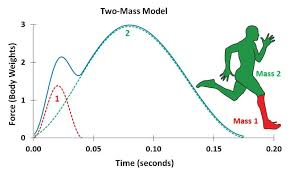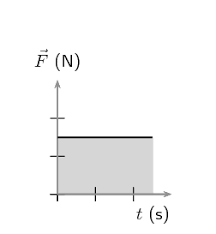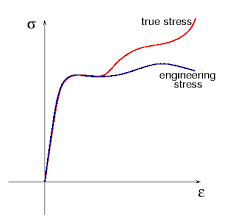## How to Calculate and Solve for Force | MotionThe image above represents force.

To compute for force, two essential parameters are needed and these parameters are mass (m) and acceleration (a).

The formula for calculating force:

F = ma

Where;

F = Force
m = Mass
a = Acceleration

Let’s solve an example;
Find the force when the mass is 12 and the acceleration is 26?

This implies that;

m = Mass = 12
a = Acceleration = 26

F = ma
F = 12 x 26
F = 312

Therefore, the force is 312 N.

Calculating for Mass when the Force and the Acceleration is Given.

m = F / a

Where;

m = Mass
F = Force
a = Acceleration

Let’s solve an example;
Find the mass when the force is 100 and the acceleration is 20.

This implies that;

F = Force = 100
a = Acceleration = 20

m = F / a
m = 100 / 20
m = 5

Therefore, the mass is 5.

## How to Calculate and Solve for Impulse | MotionThe image above represents impulse.

To compute for impulse, two essential parameters are needed and these parameters are force (f) and time (t).

The formula for calculating impulse:

I = Ft

Where;

I = Impulse
F = Force
t = Time

Let’s solve an example;
Given that the force is 28 and the time is 14. Find the impulse?

This implies that;

F = Force = 28
t = Time = 14

I = Ft
I = 28 x 14
I = 392

Therefore, the impulse is 392 Ns.

Calculating the Force when the Impulse and the Time is Given.

F = I / t

Where;

F = Force
I = Impulse
t = Time

Let’s solve an example;
Given that the impulse is 60 and the time is 10. Find the force?

This implies that;

I = Impulse = 60
t = Time = 10

F = I / t
F = 60 / 10
F = 6

Therefore, the force is 6 N.

## How to Calculate and Solve for True Stress | The Calculator EncyclopediaThe image above represents the true stress.

To compute for the true stress, two essential parameters are needed and these parameters are force (F) and instantaneous area (Ai).

The formula for calculating true stress:

σT = F / Ai

Where;
T = True Stress
F = Force
Ai = Instantaneous Area

Let’s solve an example;
Find the true stress when the instantaneous area is 60 with a force of 25.

This implies that;
F = Force = 25
Ai = Instantaneous Area = 60

σT = F / Ai
σT = 25 / 60
σT = 0.416

Therefore, the true stress is 0.416 Pa.

Calculating the Force when True Stress and Instantaneous Area is Given.

F = Ai x σT

Where;
F = Force
σT = True Stress
Ai = Instantaneous Area

Let’s solve an example;
Find the force when the instantaneous area is 30 with a true stress of 15.

This implies that;
σT = True Stress = 15
Ai = Instantaneous Area = 30

F = Ai x σT
F = 30 x 15
F = 450

Therefore, the force is 450.

## How to Convert From One Unit of Force to Another Unit of Force

Nickzom Calculator is a calculator encyclopedia tool that converts units of force. There is a large number of force units and the units that can be converted using Nickzom Calculator are:

• Newtons
• Kilonewtons
• Gram-Forces
• Kilogram-Forces
• Ton-Forces (metric)
• Exanewtons
• Petanewtons
• Teranewtons
• Giganewtons
• Meganewtons
• Hectonewtons
• Dekanewtons
• Decinewtons
• Centinewtons
• Millinewtons
• Micronewtons
• Nanonewtons
• Piconewtons
• Femtonewtons
• Attonewtons
• Dynes
• Joules/meter
• Joules/centimeter
• Ton-Forces (Short)
• Ton-Forces (Long)
• Kip-Forces
• Kilopound-Forces
• Pound-Forces
• Ounces-Forces
• Poiundals
• Pound Feet/Sq. Second
• Ponds
• Kiloponds

Let’s convert 20 Ton-Forces (metric) to Pound-Forces.